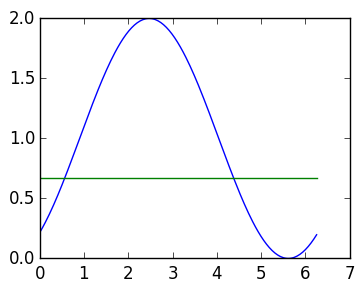# Lecture 37: Time-evolution PDEs with periodic boundary conditions¶

This lecture investigates solving time-evolution PDEs with periodic boundary conditions. We will accomplish this initially using a semi-discretization: we discretize in space, and evolve in time exactly, using the matrix exponential.

We will consider two examples.

1. The transport equation $$u_t = u_\theta, u(0,\theta)=u_0(\theta)$$ which has exact solution: $u(t,\theta) = u_0(t+\theta)$. We will use this to compare our numerical approximation.

2. Heat equation $$u_t = u_{\theta\theta}, u(0,\theta)=u_0(\theta).$$

## Discrete derivatives for periodic functions¶

We first construct discrete derivatives that incorporate boundary conditions. The trapezoidal approximation will now be 2π periodic. For example, consider the approximate $\cos(\cos(\theta))$ at $\theta_0,\ldots,\theta_n$:

In :
n=5
θ=linspace(0,2π,n+1)
plot(θ,cos(cos(θ)));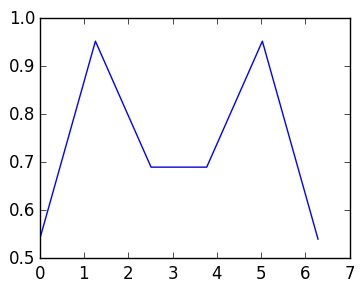Because $\cos\cos(\theta))$ is 2π periodic, we can extend it to the whole real line, as well as the piecewise affine approximation:

In :
n=5
θ=linspace(0,2π,n+1)
plot(θ,cos(cos(θ)));
plot(θ-4π,cos(cos(θ-4π)));
plot(θ-2π,cos(cos(θ-2π)));
plot(θ+2π,cos(cos(θ+2π)));
plot(θ+4π,cos(cos(θ+2π)));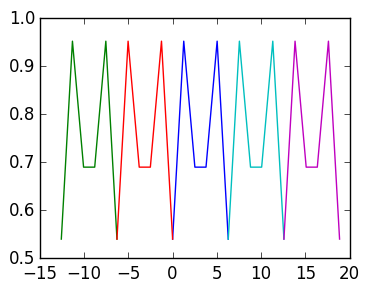That way, we can also extend the derivative:

In :
n=5
θ=linspace(0,2π,n+1)
h=1/n
dv=diff(cos(cos(θ)))/h
for k=1:length(dv)
plot([θ[k],θ[k+1]],[dv[k],dv[k]])
end

for k=length(dv)
plot([θ[k]-2π,θ[k+1]-2π],[dv[k],dv[k]])
end

for k=1
plot([θ[k]+2π,θ[k+1]+2π],[dv[k],dv[k]])
end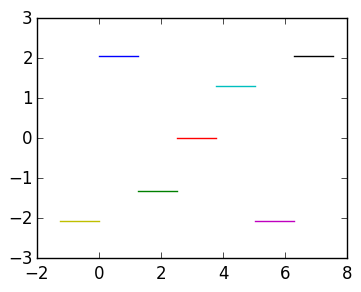Thus if we use the left-hand discretization, that is, evaluate at $\theta_0+0,\ldots,\theta_{n-1}+0$, we wrap around. This gives us the $n \times n$ discrete derivative

$$D_n^L : {\hbox{Values at } \atop \theta_0,\ldots,\theta_{n-1}} \rightarrow {\hbox{Values at } \atop \theta_0,\ldots,\theta_{n-1}}$$

Defined by

$$D_n^L \triangleq {1 \over h} \begin{pmatrix} -1 & 1 \cr &-1 & 1 \cr &&\ddots & \ddots \cr &&&-1 & 1 \cr 1 &&&&-1\end{pmatrix}$$

We define this matrix as follows:

In :
using PyPlot

function DL(n)
h=2π/n
ret=zeros(n,n)
for k=1:n-1
ret[k,k]=-1
ret[k,k+1]=1
end
ret[n,1]=1
ret[n,n]=-1
ret/h
end

n=1000
θ=linspace(0.,2π*(1-1/n),n)
norm(DL(n)*cos(θ)+sin(θ),Inf)

Out:
0.003141582318193059

An equally valid construction is to evaluate at $\theta_0-0,\ldots,\theta_{n-1}-0$, in which case we get

$$D_n^R : {\hbox{Values at } \atop \theta_0,\ldots,\theta_{n-1}} \rightarrow {\hbox{Values at } \atop \theta_0,\ldots,\theta_{n-1}}$$

defined by

$$D_n^R \triangleq {1 \over h} \begin{pmatrix} 1 &&&& -1 \cr -1 & 1 \cr &-1 & 1 \cr &&\ddots & \ddots \cr &&&-1 & 1 \end{pmatrix}$$
In :
function DR(n)
h=2π/n
ret=zeros(n,n)
for k=2:n
ret[k,k]=1
ret[k,k-1]=-1
end
ret[1,n]=-1
ret[1,1]=1
ret/h
end

n=1000
θ=linspace(0.,2π*(1-1/n),n)
norm(DR(n)*cos(θ)+sin(θ),Inf)

Out:
0.003141582318193381

## Solving PDEs with left-hand rule¶

Consider the transport equartion

$$u_t = u_\theta$$

We discretize the $\theta$-derivative using $D_n^L$, to obtain a time-dependent vector

$$\mathbf w(t) \approx \begin{pmatrix} u(t,\theta_0)\cr u(t,\theta_1) \cr \vdots \cr u(t,\theta_{n-1})\end{pmatrix}$$

that solves the ODE

$$\mathbf w'(t) = D_n^L \mathbf w(t)$$

with initial condition

$$\mathbf w(0) = \mathbf w_0 \triangleq \begin{pmatrix} u_0(\theta_0)\cr u_0(\theta_1) \cr \vdots \cr u_0(\theta_{n-1})\end{pmatrix}$$

The exact solution is given by the matrix exponential $e^{D_n^L t} \mathbf w_0$.

We can verify that this approximation converges:

In :
u0=θ->cos(θ-0.9)

n=1000

θ=linspace(0.,2π*(1-1/n),n)
w0=u0(θ)
t=1.
w1=expm(DL(n)*t)*w0

plot(θ,w1)
plot(θ,u0(t+θ))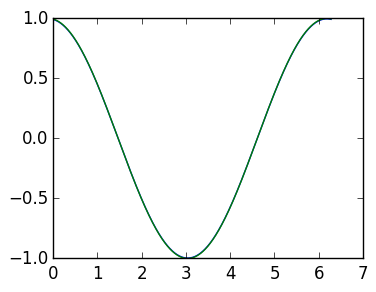Out:
1-element Array{Any,1}:
PyObject <matplotlib.lines.Line2D object at 0x30a189b90>

## Solving PDEs with right-hand rule¶

We can also discretize the equation with $D_n^R:$

$$\mathbf w'(t) = D_n^R \mathbf w(t)$$

with initial condition

$$\mathbf w(0) = \mathbf w_0 \triangleq \begin{pmatrix} u_0(\theta_0)\cr u_0(\theta_1) \cr \vdots \cr u_0(\theta_{n-1})\end{pmatrix}$$

The exact solution is given by the matrix exponential $e^{D_n^R t} \mathbf w_0$. Unfortunately, it blows up and does not approximate the true solution:

In :
u0=θ->cos(θ-0.9)

n=200
θ=linspace(0.,2π*(1-1/n),n)
w0=u0(θ)
t=1.
w1=expm(DR(n)*t)*w0
plot(θ,w1)
plot(θ,u0(t+θ))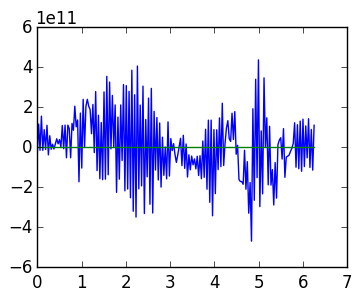Out:
1-element Array{Any,1}:
PyObject <matplotlib.lines.Line2D object at 0x321381310>

## Stability of time evolution¶

This phenomenon emphasizes the subtly of numerically solving time-evolution PDEs: getting a discretization "wrong" can have drastic effects. To understand what makes a "good" discretization, recall that, if $A=V \Lambda V^{-1}$, then

$$e^{At} = V e^{\Lambda t} V^{-1} = V \begin{pmatrix} e^{\lambda_1 t} \cr & \ddots \cr && e^{\lambda_n t} \end{pmatrix} V^{-1}.$$

Recall further that if $\lambda = q + i r$, then

$$e^{\lambda} = e^q e^{i r}.$$

Thus if $\lambda$ has positive real part, we get exponential increase and instability, and if $\lambda$ has negative real part, we have exponential decrease and stability.

Indeed, the eigenvalues of $D_n^L$ are all in the left-hand plane and so $e^{D_n^L t}$ is stable:

In :
n=100
λ=eigvals(DL(n))
scatter(real(λ),imag(λ));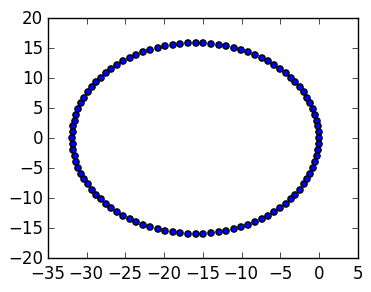While the eigenvalues of $D_n^R$ are in the right-hand plane and so $e^{D_n^Rt} is unstable: In : λ=eigvals(DR(n)) scatter(real(λ),imag(λ));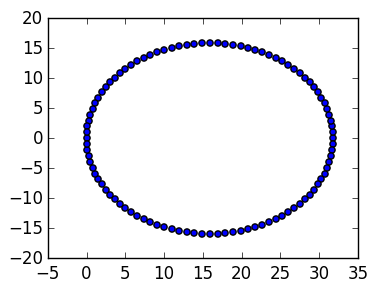Excercise How would you discretize the PDE$u_t + u_{\theta} = 0$? ## Heat equation¶ Let's now consider the heat equation: $$u_t = u_{\theta\theta}$$ We have several choices for discretizing the second derivative: 1.$(D_n^L)^2$2.$(D_n^R)^2$3.$D_n^RD_n^L$4.$D_n^LD_n^R$Inspecting the eigenvalues, we see that 3 (and 4) are stable: In : n=10 D2=DL(n)*DR(n) λ=eigvals(D2) scatter(real(λ),imag(λ));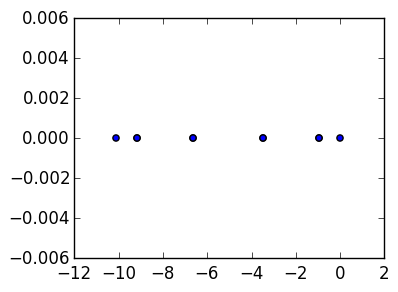Exercise Are 1 and 2 stable or unstable? We can therefore approximate the solution by $$e^{\tilde D_n^2 t} \mathbf w_0$$ where$\tilde D_n^2 = D_n^LD_n^R\$.

In :
u0=θ->sin(θ-0.9)+1

n=200

D2=DL(n)*DR(n)

θ=linspace(0.,2π*(1-1/n),n)
w0=u0(θ)
t=1.
w1=expm(D2*t)*w0
t=2.
w2=expm(D2*t)*w0

plot(θ,w0)
plot(θ,w1)
plot(θ,w2);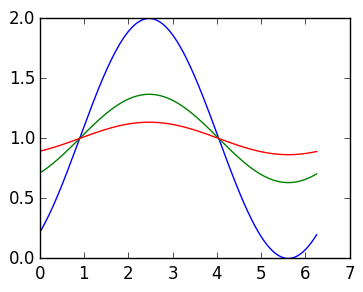If we investigate the derivatives closely, we observe that all eigenvalues are negative:

In :
eigvals(D2)

Out:
200-element Array{Float64,1}:
-4052.85
-4051.85
-4051.85
-4048.85
-4048.85
-4043.85
-4043.85
-4036.87
-4036.87
-4027.9
-4027.9
-4016.95
-4016.95
⋮
-35.8935
-24.9486
-24.9486
-15.979
-15.979
-8.99334
-8.99334
-3.99868
-3.99868
-0.999918
-0.999918
-2.52476e-14

Thus, eventually, we have artificial decay in the solution. To see this reliably, we have to implement expm ourselves using eigenvalue decomposition:

In :
t=3000000000000.

λ,Q=eig(D2)
w=Q*diagm(exp(λ*t))*Q'*w0
plot(θ,w0)
plot(θ,w)   # should tend to 1, but some decay is introduced because   -2.52476e-14 < 0.;